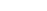[ back to Tom 7 Radar ]

w
e
b

l
i
n
k
Dividing and Multiplying by Zero (07 Apr 2003 at 19:48)
I enjoyed this transcript of a ranting lunatic math/physics hobbyist in the Australian Supreme Court. This guy is verbose beyond all reason, and apparently it all comes down to his theory of "two sets of dividing and multiplying by zero." You can also see his home page for some more honest-to-goodness pseudoscience.c
o
m
m
e
n
t
Marc (pool-68-160-180-226.bos.east.verizon.net) – 04.08.03 10:33:53
Aww jeez, I never knew court was so hilarious. Now I wanna get called for jury duty...
c
o
m
m
e
n
t
Tom 7 (gs82.sp.cs.cmu.edu) – 04.08.03 15:59:50
You'll have to work on your freestyle mathematics in preparation...
c
o
m
m
e
n
t
Anonymous (213.255.104.225) – 04.09.03 14:16:47

http://www.lalista.org/forum/viewtopic.php?t=183&postdays=0&postorder=asc&start=0
c
o
m
m
e
n
t
FARINA00 (213.255.104.225) – 04.09.03 14:17:12
Ahem, "Anonymous" being me, FARINA00. Hooray.
c
o
m
m
e
n
t
Tom 7 (h-66-167-56-44.phlapafg.covad.net) – 04.12.03 11:28:32
I'm down with "refuse real numbers" but for different reasons. ;)
c
o
m
m
e
n
t
FARINA00 (213.255.104.225) – 04.14.03 12:22:52
Hee! Tell me, tell me more
c
o
m
m
e
n
t
Tom 7 (gs82.sp.cs.cmu.edu) – 04.16.03 16:28:49
Well, classical mathematicians think of all real numbers (meaning an infinite sequence of digits after the decimal place) as existing, but you can "define" real numbers that are impossible to compute.

For instance, you can give each of the infinite number of computer programs (or turing machine) a unique number. Then, you can define the real number to be:

0.d1 d2 d3 d4 d5 ...

where d1 = 1 if the 1st computer program halts, or 0 otherwise.

Since it's undecidable whether a computer program will halt or not (search for "halting problem" on google), it's impossible to know what some of the digits of this number are.

If you can define something but never compute what it is, in what sense does that thing exist? So I refuse the existence of non-computable reals, and instead only worry about the subset that is computable. (You can think of these as infinite sequences of converging rationals, or computer programs that can produce number to an arbitrary precision.)

Interestingly, the computable reals are in one-to-one correspondence with the natural numbers, because their identity is the program that computes them. (As opposed to the "real" numbers which are proved to be bigger by diagonalization). On the other hand, testing equality of two computable reals is itself undecidable.

c
o
m
m
e
n
t
FARINA00 (213.255.104.225) – 04.17.03 07:46:46
"The determination of whether a Turing machine will come to a halt given a particular input program. The halting problem is solvable for machines with less than four states. However, the four-state case is open, and the five-state case is almost certainly unsolvable due to the fact that it includes machines iterating Collatz-like congruential functions, and such specific problems are currently open. The problem of whether a general Turing machine halts is undecidable, as first proved by Turing (Wolfram 2002, pp. 1137-1138)"
...

So, the kind of number you described, may one day be computable, assumed that the N-state case has been solved (with N=the highest state among the infinite programs making up your number).

A (subtler?) easiest, and certainly never computable example may be:

0.XY

where X=1 if Y=0
and Y=1 if X=0

This is a case I've been thinking to some time ago, criticizing the existance of certains real numbers exactly the way you did. Such a question was brought up, I believe, while writing a function that built numbers like this:

f(N)=0.XAYBCD

So that X = first prime factor and A = power
Y = second prime factor and B = power

and so on.
Bah. I hate numbers.
c
o
m
m
e
n
t
Tom 7 (gs82.sp.cs.cmu.edu) – 04.22.03 14:44:06
Well, the number can never be computed regardless of how good our ability to solve limited-state turing machines is, but as you say it may be possible to approximate it. It's easy to make numbers that are not even approximatable, like the probability that any turing machine chosen randomly (not that I believe uniform randomness over an infinite set, anyway) will halt.

There's no need to hate numbers! Just believe in what you want to believe in, and think of the rest as just symbol manipulation.p
o
s
t

a

c
o
m
m
e
n
tPlease enter the code, unless you are spammer!!